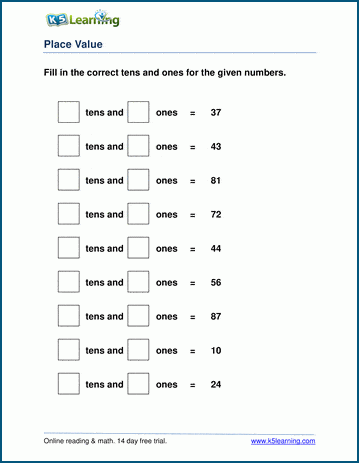# Place Value Worksheets

## Place value and base 10 blocks worksheets

Our place value worksheets focus deepening a student's understanding of our base 10 system.  In our "base 10 blocks" worksheets, students manipulate blocks (units of 1) and rods (groups of 10) to build, deconstruct or add numbers. Later worksheets focus on building 3 digit to 6 digit numbers.

Grade 1: Base 10 blocks worksheets

Topics include:

## Grade 1 base 10 blocks worksheets

• Regrouping unit blocks into blocks of 10 ("rods")
• Counting "tens" and "ones"
• Breaking a number (11-99) into rods ("tens") and  blocks ("ones")
• Adding 2 digit numbers with base 10 blocks

## Grade 1 place value worksheets

• Identifying tens and ones from 2 digit numbers
• Combining tens and ones into 2 digit numbers
• Identifying a digit's place value (tens, ones)
• Building a 2 digit number with missing addends
• Write 2 digit numbers in expanded form
• Write 2 digit numbers in normal form

## Grade 2 place value worksheets

• Building a 3-digit number from the parts
• Missing place values in 3-digit numbers
• Write 3-digit numbers in expanded form
• Write 3-digit numbers in normal form
• Hundreds, tens & ones - identify the underlined digit
• Comparing and ordering numbers up to 100 and 1,000

## Grade 3 place value worksheets

• Building 3, 4 and 5-digit numbers from the parts
• Missing place values in 3 and 4-digit numbers
• Write 4-digit numbers in expanded form
• Write 4-digit numbers in normal form
• Identify the place value of the underlined digit
• Compare and order numbers up to 10,000 and 100,000

## Grade 4 place value worksheets

• Building 4, 5 and 6-digit numbers from the parts
• Missing place values in 4, 5 and 6-digit numbers
• Write 5-digit numbers in expanded form
• Write 5-digit numbers in normal form

## Grade 5 place value worksheets

• Building 5 and 6-digit numbers from the parts
• Missing place values in 5 and 6-digit numbers
• Build a 5-digit number from parts with decimals
• Build a 6-digit number from parts with decimals

## Related topics

Comparing numbers worksheets

Rounding worksheetsSample Place Value Worksheet

What is K5?

K5 Learning offers free worksheets, flashcards and inexpensive workbooks for kids in kindergarten to grade 5. Become a member to access additional content and skip ads.